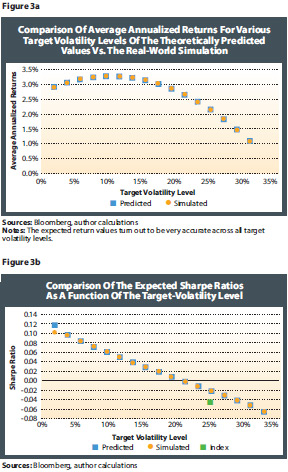Optimal Design Of Risk Control Strategy Indexes

August 17, 2012

Figure 2 also demonstrates that the expected Sharpe ratio is a linearly decreasing function of the target volatility level that is located above the Sharpe ratio of the underlying equity index. In other words, a target volatility index with the target level chosen to equal the average level of volatility of the underlying equity index always has a better Sharpe ratio than the equity index in the long run.

Even for target volatility levels chosen above the average volatility of the underlying index, the Sharpe ratio is T*.

In essence, the theoretical results suggest that any target volatility strategy index based on any equity index creates alpha from a risk/return perspective, i.e., it has a better long-run Sharpe ratio, as long as the target volatility T*.

This is a very strong result, as it implies that investors in equity-based ETFs are always better off in the long run investing in the corresponding target volatility ETF instead of the pure equity ETF.

As for the leveraged indexes, it is important to verify these theoretical results by using real-world data. As a test case, we use the Euro Stoxx 50 Index and the EONIA money market rate as portfolio components. We compare the theoretical predictions for the expected return and expected Sharpe ratio detailed in the online appendixes and illustrated in Figure 2 to the actual simulation of the corresponding target volatility strategy using the index formulas (1) and (6). Figure 3 shows the expected returns and Sharpe ratios for a variety of target volatility levels T. The simulated values turn out to be very close to the predicted values across all target volatility levels for both expected returns and Sharpe ratios.In fact, the reason the heavy-tailed nature of equity return does not have a significant influence on our analysis is the results of our analysis in comparing long-term results, i.e., returns and Sharpe ratio over a period of several years. The influence of extreme adverse moves of equity markets, which appear occasionally due to the heavy tail of equity returns, get averaged out in the long run. In the short run, however, they can lead to significant differences between predicted and actual levels of expected returns and Sharpe ratios.